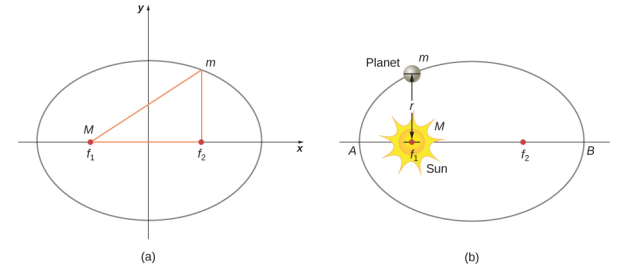$$\require{cancel}$$

# 13.6: Kepler's Laws of Planetary Motion

•• Contributed by OpenStax
• General Physics at OpenStax CNXFigure $$\PageIndex{6}$$, and many other interesting animations as well, at the School of Physics (University of New South Wales) site.

# Kepler's Third Law

Kepler’s third law states that the square of the period is proportional to the cube of the semi-major axis of the orbit. In Satellite Orbits and Energy, we derived Kepler’s third law for the special case of a circular orbit. Equation \ref{13.8} gives us the period of a circular orbit of radius r about Earth:

$$T = 2 \pi \sqrt{\frac{r^{3}}{GM_{E}}} \ldotp$$

For an ellipse, recall that the semi-major axis is one-half the sum of the perihelion and the aphelion. For a circular orbit, the semi-major axis (a) is the same as the radius for the orbit. In fact, Equation \ref{13.8} gives us Kepler’s third law if we simply replace r with a and square both sides.

$$T^{2} = \frac{4 \pi^{2}}{GM} a^{3} \label{13.11}$$

We have changed the mass of Earth to the more general M, since this equation applies to satellites orbiting any large mass.

Example $$\PageIndex{1}$$: Orbit of Halley’s Comet

Determine the semi-major axis of the orbit of Halley’s comet, given that it arrives at perihelion every 75.3 years. If the perihelion is 0.586 AU, what is the aphelion?

Strategy

We are given the period, so we can rearrange Equation \ref{13.11}, solving for the semi-major axis. Since we know the value for the perihelion, we can use the definition of the semi-major axis, given earlier in this section, to find the aphelion. We note that 1 Astronomical Unit (AU) is the average radius of Earth’s orbit and is defined to be 1 AU = 1.50 x 1011 m.

Solution

Rearranging Equation 13.11 and inserting the values of the period of Halley’s comet and the mass of the Sun, we have

$$\begin{split} a & = \left(\dfrac{GM}{4 \pi^{2}} T^{2}\right)^{1/3} \\ & = \left(\dfrac{(6.67 \times 10^{-11}\; N\; \cdotp m^{2}/kg^{2})(2.00 \times 10^{30}\; kg)}{4 \pi^{2}} (75.3\; yr \times 365\; days/yr \times 24\; hr/day \times 3600\; s/hr)^{2}\right)^{1/3} \ldotp \end{split}$$

This yields a value of 2.67 x 1012 m or 17.8 AU for the semi-major axis. The semi-major axis is one-half the sum of the aphelion and perihelion, so we have

$$\begin{split} a & = \frac{1}{2} (aphelion + perihelion) \\ aphelion & = 2a - perihelion \ldotp \end{split}$$

Substituting for the values, we found for the semi-major axis and the value given for the perihelion, we find the value of the aphelion to be 35.0 AU.

Significance

Edmond Halley, a contemporary of Newton, first suspected that three comets, reported in 1531, 1607, and 1682, were actually the same comet. Before Tycho Brahe made measurements of comets, it was believed that they were one-time events, perhaps disturbances in the atmosphere, and that they were not affected by the Sun. Halley used Newton’s new mechanics to predict his namesake comet’s return in 1758.

Exercises $$\PageIndex{1}$$

The nearly circular orbit of Saturn has an average radius of about 9.5 AU and has a period of 30 years, whereas Uranus averages about 19 AU and has a period of 84 years. Is this consistent with our results for Halley’s comet?

# Contributors

• Samuel J. Ling (Truman State University), Jeff Sanny (Loyola Marymount University), and Bill Moebs with many contributing authors. This work is licensed by OpenStax University Physics under a Creative Commons Attribution License (by 4.0).